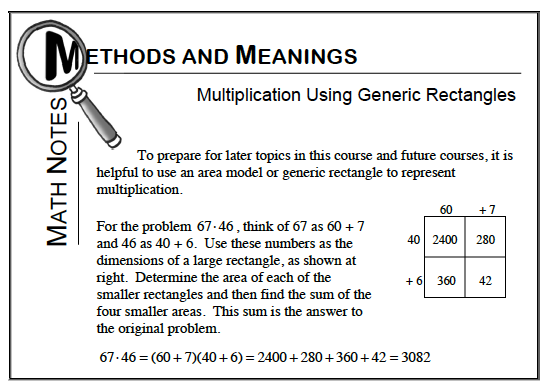### Home > CC1 > Chapter 6 > Lesson 6.1.4 > Problem6-58

6-58.

1.  $+6$ $20$ $1600$ $560$
1.  $+5$ $30$ $2700$ $3600$ $45$